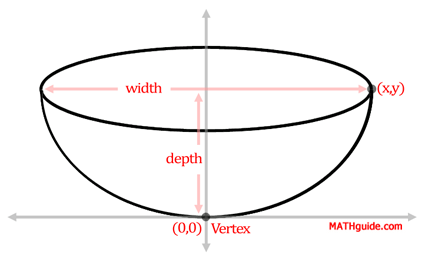Problem: A satelite dish (paraboloid) has a 2 foot width and a 4 foot depth. Determine the equation of a two-dimensional cross section of this paraboloid.   Round answers to the nearest tenth.Location of (x,y): (, ) Equation: x2 = y Receiver Location: The receiver should be placed  feet above the vertex.